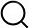# Also in the Article

EIS characterization of the (Au) electrode-PEDOT:PSS system

Procedure

An equivalent circuit model of the (Au) electrode-PEDOT:PSS system was developed (Fig. 2A) using electrochemical measurements. In this model, the finite-length Warburgh diffusion impedance (ZWarburg) is defined as (45)$ZWarburg=1Y0jωcoth(jωτD)1/2$(2)where ω is the frequency and Y0 is the frequency-independent admittance Y0 = CDD)−1/2. Electrical impedance spectroscopy (EIS) measurements were used to find the circuit parameters. Impedance spectra were recorded by applying a sinusoidal excitation signal with an excitation amplitude of 10 mV (rms) that is superimposed on a constant dc potential of 200 mV. Frequency-dependent electronic response of the Au electrode-PEDOT:PSS system was fitted to an equivalent circuit model. Circuit parameters were obtained using Echem Analyst software.

Note: The content above has been extracted from a research article, so it may not display correctly.

Q&A